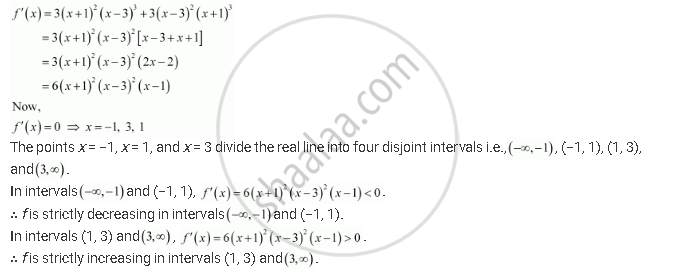Share

# Find the Intervals in Which the Following Functions Are Strictly Increasing Or Decreasing: (X + 1)3 (X − 3)3 - CBSE (Science) Class 12 - Mathematics

ConceptIncreasing and Decreasing Functions

#### Question

Find the intervals in which the following functions are strictly increasing or decreasing:

(x + 1)3 (x − 3)3

#### Solution

We have,

f(x) = (x + 1)3 (x − 3)3Is there an error in this question or solution?

#### APPEARS IN

NCERT Solution for Mathematics Textbook for Class 12 (2018 to Current)
Chapter 6: Application of Derivatives
Q: 6.5 | Page no. 205

#### Video TutorialsVIEW ALL 

Solution Find the Intervals in Which the Following Functions Are Strictly Increasing Or Decreasing: (X + 1)3 (X − 3)3 Concept: Increasing and Decreasing Functions.
S